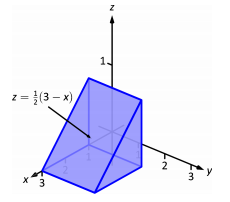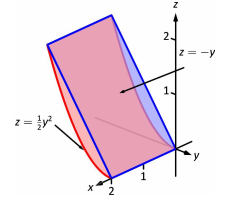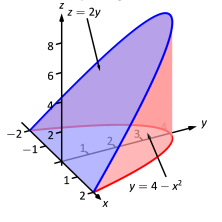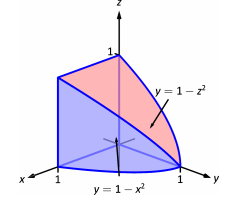$$\newcommand{\id}{\mathrm{id}}$$ $$\newcommand{\Span}{\mathrm{span}}$$ $$\newcommand{\kernel}{\mathrm{null}\,}$$ $$\newcommand{\range}{\mathrm{range}\,}$$ $$\newcommand{\RealPart}{\mathrm{Re}}$$ $$\newcommand{\ImaginaryPart}{\mathrm{Im}}$$ $$\newcommand{\Argument}{\mathrm{Arg}}$$ $$\newcommand{\norm}{\| #1 \|}$$ $$\newcommand{\inner}{\langle #1, #2 \rangle}$$ $$\newcommand{\Span}{\mathrm{span}}$$

# 14.4E: Triple Integrals (Exercises)

$$\newcommand{\vecs}{\overset { \rightharpoonup} {\mathbf{#1}} }$$ $$\newcommand{\vecd}{\overset{-\!-\!\rightharpoonup}{\vphantom{a}\smash {#1}}}$$$$\newcommand{\id}{\mathrm{id}}$$ $$\newcommand{\Span}{\mathrm{span}}$$ $$\newcommand{\kernel}{\mathrm{null}\,}$$ $$\newcommand{\range}{\mathrm{range}\,}$$ $$\newcommand{\RealPart}{\mathrm{Re}}$$ $$\newcommand{\ImaginaryPart}{\mathrm{Im}}$$ $$\newcommand{\Argument}{\mathrm{Arg}}$$ $$\newcommand{\norm}{\| #1 \|}$$ $$\newcommand{\inner}{\langle #1, #2 \rangle}$$ $$\newcommand{\Span}{\mathrm{span}}$$ $$\newcommand{\id}{\mathrm{id}}$$ $$\newcommand{\Span}{\mathrm{span}}$$ $$\newcommand{\kernel}{\mathrm{null}\,}$$ $$\newcommand{\range}{\mathrm{range}\,}$$ $$\newcommand{\RealPart}{\mathrm{Re}}$$ $$\newcommand{\ImaginaryPart}{\mathrm{Im}}$$ $$\newcommand{\Argument}{\mathrm{Arg}}$$ $$\newcommand{\norm}{\| #1 \|}$$ $$\newcommand{\inner}{\langle #1, #2 \rangle}$$ $$\newcommand{\Span}{\mathrm{span}}$$

## Terms and Concepts

1. The strategy for establishing bounds for triple integrals is "from ________ to ________, then from ________ to ________ and then from ________ to ________."

We integrate from surface to surface, then from curve to curve and then from point to point.

2. Give an informal interpretation of what $$\displaystyle \iiint_Q \,dV$$ means.

$$\displaystyle \iiint_Q \,dV$$ = Volume of the solid region $$Q$$

3. Give two uses of triple integration.

To compute total mass or average density of a solid object, given a density function or to compute the average temperature in a solid region or object.

4. If an object has a constant density $$\delta$$ and a volume $$V$$, what is its mass?

It's mass is $$\delta V$$.

## Volume of Solid Regions

In Exercises 5-8, two surfaces $$f_1(x,y)$$ and $$f_2(x,y)$$ and a region $$R$$ in the $$xy$$-plane are given. Set up and evaluate the triple integral that represents the volume between these surfaces over $$R$$.

5. $$f_1(x,y) = 8-x^2-y^2,\,f_2(x,y) =2x+y;$$
$$R$$ is the square with corners $$(-1,-1)$$ and $$(1,1)$$.

V = $$\displaystyle \int_{-1}^{1}\int_{-1}^{1}\int_{2x+y}^{8-x^2-y^2} \,dz\,dy\,dx\quad$$ $$=\quad\dfrac{88}{3}\,\text{units}^3$$

6. $$f_1(x,y) = x^2+y^2,\,f_2(x,y) =-x^2-y^2;$$
$$R$$ is the square with corners $$(0,0)$$ and $$(2,3)$$.

7. $$f_1(x,y) = \sin x \cos y,\,f_2(x,y) =\cos x \sin y +2;$$
$$R$$ is the triangle with corners $$(0,0), \,(\pi , 0)$$ and $$(\pi,\pi)$$.

V = $$\displaystyle \int_{0}^{\pi}\int_{0}^{x}\int_{\sin x\cos y}^{\cos x\sin y + 2} \,dz\,dy\,dx\quad$$ $$=\quad\left(\pi^2 - \pi\right)\,\text{units}^3\quad$$ $$\approx 6.72801\,\text{units}^3$$

8. $$f_1(x,y) = 2x^2+2y^2+3,\,f_2(x,y) =6-x^2-y^2;$$
$$R$$ is the circle $$x^2+y^2=1$$.

In Exercises 9-16, a domain $$D$$ is described by its bounding surfaces, along with a graph. Set up the triple integral that gives the volume of $$D$$ in the indicated order(s) of integration, and evaluate the triple integral to find this volume.

9. $$D$$ is bounded by the coordinate planes and $$z=2-\frac{2}{3}x-2y$$.
Evaluate the triple integral with order $$dz\,dy\,dx$$.V = $$\displaystyle \int_{0}^{3}\int_{0}^{1-\frac{x}{3}}\int_{0}^{2 - \frac{2}{3}x-2y} \,dz\,dy\,dx\quad$$ $$=\quad 1\,\text{unit}^3$$

10. $$D$$ is bounded by the planes $$y=0,y=2,x=1,z=0$$ and $$z=(2-x)/2$$.
Evaluate the triple integral with order $$dx\,dy\,dz$$.11. $$D$$ is bounded by the planes $$x=0,x=2,z=-y$$ and by $$z=y^2/2$$.
Evaluate the triple integral with orders $$dy\,dz\,dx$$ and $$dz\,dy\,dx$$ to verify that you obtain the same volume either way.V = $$\displaystyle \int_{0}^{2}\int_{0}^{2}\int_{-\sqrt{2z}}^{-z} \,dy\,dz\,dx\quad$$ $$=\quad \dfrac{4}{3}\,\text{unit}^3$$
V = $$\displaystyle \int_{0}^{2}\int_{-2}^{0}\int_{\frac{y^2}{2}}^{-y} \,dz\,dy\,dx\quad$$ $$=\quad \dfrac{4}{3}\,\text{unit}^3$$

12. $$D$$ is bounded by the planes $$z=0,y=9, x=0$$ and by $$z=\sqrt{y^2-9x^2}$$.
Do not evaluate any triple integral. Just set this one up.13. $$D$$ is bounded by the planes $$x=2,y=1,z=0$$ and $$z=2x+4y-4$$.
Evaluate the triple integral with orders $$dz\,dy\,dx$$ and $$dx\,dy\,dz$$ to verify that you obtain the same volume either way.V = $$\displaystyle \int_{0}^{2}\int_{1-\frac{x}{2}}^{1}\int_{0}^{2x+4y-4} \,dz\,dy\,dx\quad$$ $$=\quad\dfrac{4}{3}\,\text{units}^3$$
V = $$\displaystyle \int_{0}^{4}\int_{\frac{z}{4}}^{1}\int_{(z-4y+4)/2}^{2} \,dx\,dy\,dz\quad$$ $$=\quad\dfrac{4}{3}\,\text{units}^3$$

14. $$D$$ is bounded by the plane $$z=2y$$ and by $$y=4-x^2$$.
Evaluate the triple integral with order $$dz\,dy\,dx$$.15. $$D$$ is bounded by the coordinate planes and $$y=1-x^2$$ and $$y=1-z^2$$.
Do not evaluate any triple integral. Which order would be easier to evaluate: $$dz\,dy\,dx$$ or $$dy\,dz\,dx$$? Explain why.V = $$\displaystyle \int_{0}^{1}\int_{0}^{1-x^2}\int_{0}^{\sqrt{1-y}} \,dz\,dy\,dx\quad$$
V = $$\displaystyle \int_{0}^{1}\int_{0}^{x}\int_{0}^{1-x^2} \,dy\,dz\,dx + \displaystyle \int_{0}^{1}\int_{x}^{1}\int_{0}^{1-z^2} \,dy\,dz\,dx$$
The first one is easier since it only requires evaluation of a single integral, although both can be evaluated fairly easily.

16. $$D$$ is bounded by the coordinate planes and by $$z=1-y/3$$ and $$z=1-x$$.
Evaluate the triple integral with order $$dx\,dy\,dz$$.## Evaluating General Triple Integrals

In exercises 17 - 20, evaluate the triple integrals over the rectangular solid box $$B$$.

17. $$\displaystyle \iiint_B (2x + 3y^2 + 4z^3) \space dV,$$ where $$B = \big\{(x,y,z) \,|\, 0 \leq x \leq 1, \space 0 \leq y \leq 2, \space 0 \leq z \leq 3\big\}$$

$$192$$

18. $$\displaystyle \iiint_B (xy + yz + xz) \space dV,$$ where $$B = \big\{(x,y,z) \,|\, 1 \leq x \leq 2, \space 0 \leq y \leq 2, \space 1 \leq z \leq 3\big\}$$

19. $$\displaystyle \iiint_B (x \space cos \space y + z) \space dV,$$ where $$B = \big\{(x,y,z) \,|\, 0 \leq x \leq 1, \space 0 \leq y \leq \pi, \space -1 \leq z \leq 1\big\}$$

$$0$$

20. $$\displaystyle \iiint_B (z \space sin \space x + y^2) \space dV,$$ where $$B = \big\{(x,y,z) \,|\, 0 \leq x \leq \pi, \space 0 \leq y \leq 1, \space -1 \leq z \leq 2\big\}$$

In Exercises 21 - 24, evaluate the triple integral.

21. $$\displaystyle \int_{-\pi/2}^{\pi/2}\int_{0}^{\pi}\int_{0}^{\pi} (\cos x \sin y \sin z )\,dz\,dy\,dx$$

$$8$$

22. $$\displaystyle \int_{0}^{1}\int_{0}^{x}\int_{0}^{x+y} (x+y+z )\,dz\,dy\,dx$$

23. $$\displaystyle \int_{0}^{\pi}\int_{0}^{1}\int_{0}^{z} (\sin (yz))\,dx\,dy\,dz$$

$$\pi$$

24. $$\displaystyle \int_{\pi}^{\pi^2}\int_{x}^{x^3}\int_{-y^2}^{y^2} (\cos x \sin y \sin z )\,dz\,dy\,dx$$

## Average Value of a Function

25. Find the average value of the function $$f(x,y,z) = x + y + z$$ over the parallelepiped determined by $$x + 0, \space x = 1, \space y = 0, \space y = 3, \space z = 0$$, and $$z = 5$$.

$$\frac{9}{2}$$

26. Find the average value of the function $$f(x,y,z) = xyz$$ over the solid $$E = [0,1] \times [0,1] \times [0,1]$$ situated in the first octant.

## Approximating Triple Integrals

27. The midpoint rule for the triple integral $$\displaystyle \iiint_B f(x,y,z) \,dV$$ over the rectangular solid box $$B$$ is a generalization of the midpoint rule for double integrals. The region $$B$$ is divided into subboxes of equal sizes and the integral is approximated by the triple Riemann sum $\sum_{i=1}^l \sum_{j=1}^m \sum_{k=1}^n f(\bar{x_i}, \bar{y_j}, \bar{z_k}) \Delta V,\nonumber$ where $$(\bar{x_i}, \bar{y_j}, \bar{z_k})$$ is the center of the box $$B_{ijk}$$ and $$\Delta V$$ is the volume of each subbox. Apply the midpoint rule to approximate $\iiint_B x^2 \,dV\nonumber$ over the solid $$B = \big\{(x,y,z) \,|\, 0 \leq x \leq 1, \space 0 \leq y \leq 1, \space 0 \leq z \leq 1 \big\}$$ by using a partition of eight cubes of equal size. Round your answer to three decimal places.

$$\displaystyle \iiint_B f(x,y,z) \,dV\quad$$ $$\approx\quad\frac{5}{16} \approx 0.313$$

28. [T] a. Apply the midpoint rule to approximate $\iiint_B e^{-x^2} \,dV\nonumber$ over the solid $$B = \{(x,y,z) | 0 \leq x \leq 1, \space 0 \leq y \leq 1, \space 0 \leq z \leq 1 \}$$ by using a partition of eight cubes of equal size. Round your answer to three decimal places.

b. Use a CAS to improve the above integral approximation in the case of a partition of $$n^3$$ cubes of equal size, where $$n = 3,4, ..., 10$$.

## Applications

29. Suppose that the temperature in degrees Celsius at a point $$(x,y,z)$$ of a solid $$E$$ bounded by the coordinate planes and the plane $$x + y + z = 5$$ is given by: $T (x,y,z) = xz + 5z + 10\nonumber$ Find the average temperature over the solid.

$$17.5^{\circ}$$ C

30. Suppose that the temperature in degrees Fahrenheit at a point $$(x,y,z)$$ of a solid $$E$$ bounded by the coordinate planes and the plane $$x + y + z = 5$$ is given by: $T(x,y,z) = x + y + xy\nonumber$ Find the average temperature over the solid.

31. If the charge density at an arbitrary point $$(x,y,z)$$ of a solid $$E$$ is given by the function $$\rho (x,y,z)$$, then the total charge inside the solid is defined as the triple integral $$\displaystyle \iiint_E \rho (x,y,z) \,dV.$$ Assume that the charge density of the solid $$E$$ enclosed by the paraboloids $$x = 5 - y^2 - z^2$$ and $$x = y^2 + z^2 - 5$$ is equal to the distance from an arbitrary point of $$E$$ to the origin. Set up the integral that gives the total charge inside the solid $$E$$.

Total Charge inside the Solid $$E \quad=\quad$$ $$\displaystyle \int_{-\sqrt{5}}^{\sqrt{5}}\int_{-\sqrt{5-y^2}}^{\sqrt{5-y^2}}\int_{y^2+z^2-5}^{5 - y^2 - z^2} \sqrt{x^2+y^2+z^2}\,dx\,dz\,dy$$
32. Show that the volume of a regular right hexagonal pyramid of edge length $$a$$ is $$\dfrac{a^3 \sqrt{3}}{2}$$ by using triple integrals.